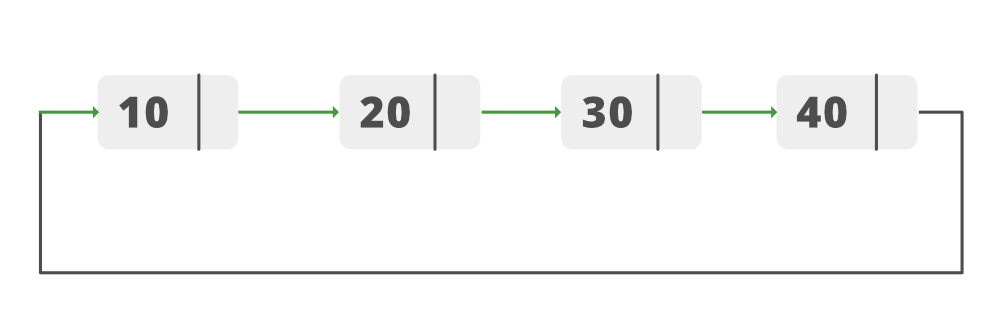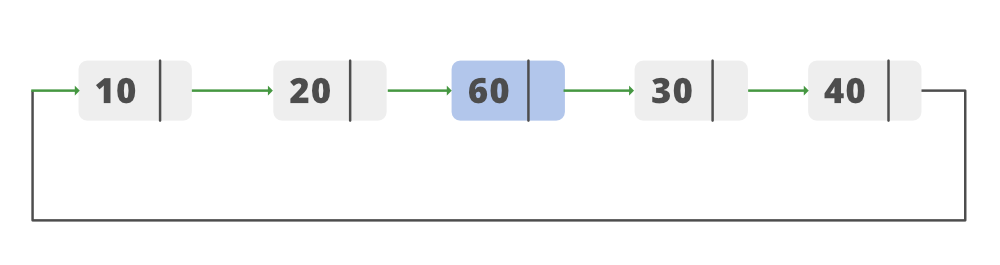# Java Program to Insert a New Node at the Middle of the Circular Linked List

• Difficulty Level : Medium
• Last Updated : 19 Jan, 2021

Given a Circular Linked List, the task is to add a New Node at the Middle of the List. Let’s consider the following Circular Linked List:List-Before-InsertionList-After-Insertion

1. Create a new node (New_node).
2. Check for an empty list. If the list is empty then insert the node as head.
3. For non-empty list, calculate the length of the list.
4. Create variable mid and store middle length in it.
5. Create two nodes Temporary and Current.
6. Now traverse the list till Temporary reaches the midpoint of the list using the mid variable.
7. Insert the New_node after the Current.
8. Make Current.next point to New node and New_node.next to Temporary.
9. Node is inserted.

Below is the implementation of the above approach:

Attention reader! Don’t stop learning now. Get hold of all the important DSA concepts with the DSA Self Paced Course at a student-friendly price and become industry ready.  To complete your preparation from learning a language to DS Algo and many more,  please refer Complete Interview Preparation Course.

In case you wish to attend live classes with experts, please refer DSA Live Classes for Working Professionals and Competitive Programming Live for Students.

## Java

 `// Java Program to Insert a New Node at``// the Middle of the Circular Linked List``import` `java.io.*;`` ` `public` `class` `GFG {``    ``// Stores Information about Node of List``    ``public` `class` `Node {``        ``int` `data;``        ``Node next;``        ``public` `Node(``int` `data) { ``this``.data = data; }``    ``}`` ` `    ``// Declaring Head of the Node``    ``public` `Node head_of_node = ``null``;`` ` `    ``// A last pointer to help append values to our list``    ``public` `Node last = ``null``;`` ` `    ``// keep count of  size of the list``    ``public` `int` `length_of_list;`` ` `    ``// Add method adds values to the end of the list``    ``public` `void` `add(``int` `data)``    ``{``        ``Node newNode = ``new` `Node(data);``        ``if` `(head_of_node == ``null``) {``            ``head_of_node = newNode;``            ``last = newNode;``            ``newNode.next = head_of_node;``        ``}``        ``else` `{``            ``last.next = newNode;``            ``last = newNode;``            ``last.next = head_of_node;``        ``}``        ``// keep count of size of list``        ``length_of_list++;``    ``}`` ` `    ``// Method to insert Node in the middle of the list``    ``public` `void` `Insert_In_Middle(``int` `data)``    ``{`` ` `        ``// creating a new node that is to be inserted``        ``Node New_node = ``new` `Node(data);`` ` `        ``// check for empty list``        ``if` `(head_of_node == ``null``) {``            ``head_of_node = New_node;``            ``last = New_node;``            ``New_node.next = head_of_node;``        ``}`` ` `        ``// If the list is not empty``        ``else` `{``            ``// Declaring nodes``            ``Node temporary;``            ``Node current;`` ` `            ``// Checking if the length of list``            ``// if even or odd`` ` `            ``int` `mid_point_check = (length_of_list % ``2``);`` ` `            ``// mid point for even length``            ``if` `(mid_point_check == ``0``) {``                ``length_of_list = length_of_list / ``2``;``            ``}``            ``// mid point for odd length``            ``else` `{``                ``length_of_list = (length_of_list + ``1``) / ``2``;``            ``}`` ` `            ``// temporary points to the head of list``            ``temporary = head_of_node;``            ``current = ``null``;``            ``// loop till we reach the mid point``            ``while` `(length_of_list > ``0``) {`` ` `                ``// make current point to the previous node``                ``current = temporary;``                ``temporary = temporary.next;``                ``length_of_list -= ``1``;``            ``}`` ` `            ``// make the previous node point to the new node``            ``current.next = New_node;`` ` `            ``//  make the new_node.next point to temporary``            ``New_node.next = temporary;``        ``}``        ``// increasing the length of list after insertion of``        ``// new node``        ``length_of_list++;``    ``}`` ` `    ``// Print_list method iterates through the list and``    ``// prints the values stored in the list``    ``public` `void` `Print_List()``    ``{``        ``Node current = head_of_node;``        ``if` `(head_of_node == ``null``) {``            ``System.out.println(``"Your list is empty"``);``        ``}``        ``else` `{``            ``do` `{``                ``System.out.print(``" "` `+ current.data);``                ``current = current.next;``            ``} ``while` `(current != head_of_node);``            ``System.out.println();``        ``}``    ``}`` ` `    ``// Driver code``    ``public` `static` `void` `main(String[] args)``    ``{`` ` `        ``GFG circular_list = ``new` `GFG();``        ``circular_list.add(``10``);``        ``circular_list.add(``20``);``        ``circular_list.add(``30``);``        ``circular_list.add(``40``);`` ` `        ``System.out.print(``"Original List        --> "``);``        ``circular_list.Print_List();``        ``circular_list.Insert_In_Middle(``60``);``        ``System.out.print(``"List after Inserting --> "``);``        ``circular_list.Print_List();``    ``}``}`
Output
```Original List        -->  10 20 30 40
List after Inserting -->  10 20 60 30 40
```

My Personal Notes arrow_drop_up